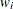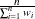WEIGHT Statement

WEIGHT variable </ option> ;

When a WEIGHT statement appears, each observation in the input data set is weighted by the value of the WEIGHT variable. Unlike a FREQ variable, the values of the WEIGHT variable can be nonintegral and are not truncated. Observations with negative, zero, or missing values for the WEIGHT variable are not used in the model fitting. When the WEIGHT statement is not specified, each observation is assigned a weight of 1. The WEIGHT statement is not available with the STRATA statement. If you specify more than one WEIGHT statement, then the first WEIGHT variable is used.

If a SCORE statement is specified, then the WEIGHT variable is used for computing fit statistics and the ROC curve, but it is not required for scoring. If the DATA= data set in the SCORE statement does not contain the WEIGHT variable, the weights are assumed to be 1 and a warning message is issued in the SAS log. If you fit a model and perform the scoring in the same run, the same WEIGHT variable is used for fitting and scoring. If you fit a model in a previous run and input it with the INMODEL= option in the current run, then the WEIGHT variable can be different from the one used in the previous run; however, if a WEIGHT variable was not specified in the previous run, you can still specify a WEIGHT variable in the current run.

Caution: PROC LOGISTIC does not compute the proper variance estimators if you are analyzing survey data and specifying the sampling weights through the WEIGHT statement. The SURVEYLOGISTIC procedure is designed to perform the necessary, and correct, computations.

The following option can be added to the WEIGHT statement after a slash (/):

NORMALIZE
NORM

causes the weights specified by the WEIGHT variable to be normalized so that they add up to the actual sample size. Weightsare normalized by multiplying them by, where n is the sample size. With this option, the estimated covariance matrix of the parameter estimators is invariant to the scale of the WEIGHT variable.